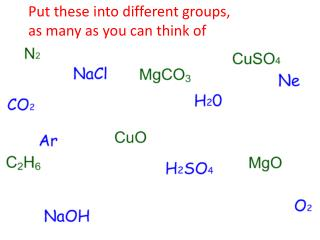DownloadDownload PresentationPut these into different groups, as many as you can think of

# Put these into different groups, as many as you can think of

Télécharger la présentation## Put these into different groups, as many as you can think of

- - - - - - - - - - - - - - - - - - - - - - - - - - - E N D - - - - - - - - - - - - - - - - - - - - - - - - - - -
##### Presentation Transcript

1. Put these into different groups, as many as you can think of

2. Groups • Compounds & elements • Green & blue • Ones that were gases & others at rm temp • Group 7 & others

3. Objectives I want you to be able to ........... • Explain why heating to constant mass is important in an experiment • Calculate the amount of moles • Write out a chemical equation correctly & balance the equation

4. Balancing Equations • Atoms are NOT created or destroyed in chemical reactions – they are REARRANGED • So equations for chemical reactions need to be balanced • Balanced means having the same number of atoms of each side

5. Steps to balance an equation • Write the reactants and products Magnesium + Oxygen = Magnesium oxide Calcium + Water = Calcium hydroxide + Hydrogen • Write the formulae for the substances; including state symbols Mg (s) + O2(g) = MgO(s) Ca (s) + H2O(l) = Ca(OH)2 (aq) + H2(g)

6. Balance the equation so that the same number of each atom are on both side. You can only add numbers in FRONT of formulae (if after then altering the substance) 2Mg(s) + O2(g)→2MgO(s) 2Mg2O→2Mg2O Ca (s) + 2H2O(l) →Ca(OH)2 (aq) + H2(g) 1Ca2H2O→1Ca2O4H

7. Magnesium sulfate crystals Epsom Salts

8. Making Epsom salts • Last lesson we made the hydrated salts. MgCO3 + H2SO4 = MgSO4 + CO2 + H2O What is missing in this formulae? MgCO3 (S) + H2SO4 (aq) = MgSO4 (s) + CO2 (g) + H2O (l) • Now will be analysing them.....

9. We did not heat to dryness, thus we have the formulae • MgSO4XH2O (s) • Read instructions on the sheet from analysing magnesium sulfate crystals through once • Follow – be careful – it will be a HOT crucible, may spit so goggles on, no sitting down etc. • 20 minutes to carry it out then complete the questions

10. Objectives I want you to be able to ........... • Explain why heating to constant mass is important in an experiment • Calculate the amount of moles • Write out a chemical equation correctly & balance the equation